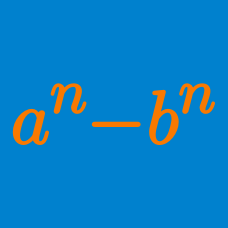Algebra

# Factorization of Polynomials

The polynomial $3x^5+13x^4+3x^3+3x^2+13x+3$ can be factorized as the product of two quadratics and one linear polynomial in $x$, all with positive integer leading coefficients. What is the sum of all of the coefficients and constant terms in the three factors?

Given that $f(x)$ and $g(x)$ are two non-constant polynomials with integer coefficients such that

$f(x) \times g(x) = x^6-6x^5+4x^4-12x^3-8,$

evaluate $\lvert f(2)+g(2) \rvert.$

Details and assumptions

You may use the fact that $f(2) \times g(2) = -168$.

For what constant $k$ can the polynomial $(x+1)(x+5)(x+9)(x+13)+k$ be factorized into a perfect square of a quadratic in $x?$

If the polynomial $x^4-9x^2+20$ is factorized as $(x+a)(x+b)(x+c)(x+d),$ where $a, b, c$ and $d$ are real numbers, what is the value of $a^2+b^2+c^2+d^2?$

Let $f(x) = x^2 - 1$. How many distinct real roots are there to $f ( f( f(x))) = 0$?

×